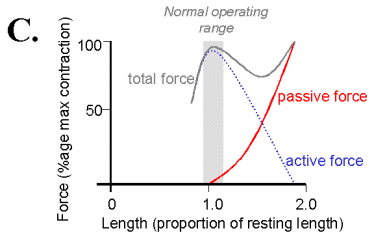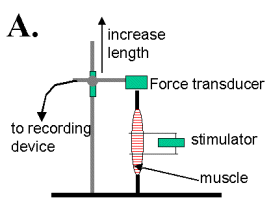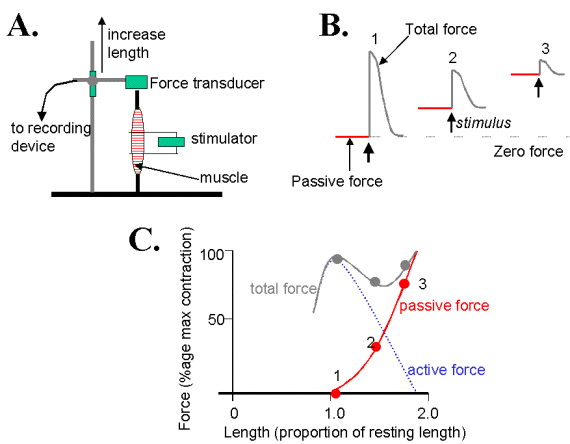Contents | 1 | 2 | Previous | Next

 1. The [muscle] length-tension relationRecap of the sliding filament theory Top

The contraction of muscle is explained by the sliding filament hypothesis that was proposed in the late 1960s by A.F. Huxley and co-workers. The contractile filaments in striated muscle are highly ordered and this gives rise to the alternate light and dark banding pattern that is characteristic of striated muscle. It turns out that this banding pattern and the relationship between muscle length & active tension in skeletal muscle were the principal features that lead Huxley and co-workers to the idea that the contraction was due to overlapping filaments that slid over one another during active shortening. The length tension relation that you may have seen in most text-books has the general form:Figure 1 - length tension relation for skeletal muscle

Notice that the units of the x-axis are 'normal resting length' so that 1.0 equates to normal operating length and 2.0 will be twice the normal length. Notice also that the normal operating range is restricted to a narrow band. In other words, your skeletal muscles never change length more than about 10 %.

The y-axis will either be 'force' with units of Newtons (or sometimes kg) or 'stress' which will have the same units but divided by area i.e. N.m-2 or kg.m-2.

You should realize then, that the length-tension relation is something that must be measured in vitro under conditions where muscle length can be altered over a wide range. The experimental setup will look something like:Figure 2 - Experimental setup required to determine length-tension relation of muscle

 Length-tension relation measured isometrically Top

The main thing to note about this experiment is that the contraction of the muscle is 'isometric' which means that contraction will occur without a change in length - the muscle does not shorten!

When the muscle is set at increasing lengths there will come a point where the muscle will resist being stretched out and a force will need to be applied to lengthen the muscle. In fact muscle is quite elastic.

So, with the muscle relaxed, there will be a force which we'll call the 'passive' force that is required to extend the muscle. If you look at figure 1 you can see how the passive force increases with muscle length and please notice that passive force only becomes appreciable at lengths greater than the normal range.

As mentioned in the lectures, one cannot directly measure active force. The experimental setup shown in figure 2. can measure passive force and 'total' force. Active force can then be calculated as total - passive. The graph in figure 1 can be hard to understand and sometimes it is helpful to see the experimental records that underlie such a graph. This is shown in the composite figure below:Figure 3 - Combined figure illustrating: A. the experimental setup, B. passive and total tension at 3 different muscle lengths & C. the length-tension data in graphical form.

Remember that the curve for active force is calculated from the curves for passive and total force!

To this point we've considered how [active ] tension varies with length where length is measured only in terms of the resting length of the muscle. Check out figure 1 if you've missed this point of principal. In the next section we will consider how tension varies as a function of the sarcomere length.

 Contents | 1 | 2 | Previous | Next